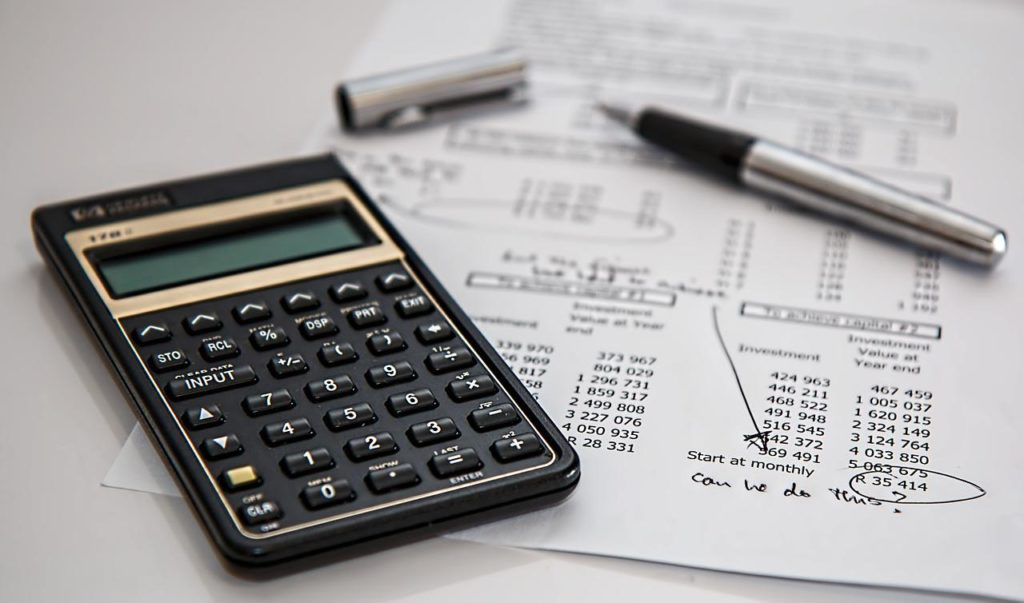# How To Calculate Cap Rate On Rental PropertyA property’s value is determined by its ability to create a certain amount of income, and a cap rate is a useful tool for doing so. If you’re a real estate investor who flips houses, the cap rate may not be as important to you as it is to other investors.

Calculating a property’s cap rate is a wonderful way for investors with rental properties in their portfolio to see the estate’s actual cash flow and worth.

## What is a cap rate?

Measurement of a rental property’s performance is done through the use of cap rates (short for capitalization rates). A cap rate is a percentage that you can use to assess the possible return on a financial asset after doing the figures.

Any investment property should have its cap rate calculated annually. If you have a large number of properties, this is extremely critical. Annually calculating cap rates will help you determine which properties are performing and which are not. There are a few things to keep in mind when acquiring a house for the first time.

Using the following formula, you may compute cap rates

• Net income is the difference between the total revenue and the total costs.
• Subtract the purchase price from the net profit.
• Calculate the percentage by moving the decimal two spaces to the right. This is the maximum rate at which you can borrow money.

## Example of a Cap Rate Calculation

You’ll first need to determine how much rent the property will bring in each year. If you’re looking to buy a rental property, you can usually find out if it was previously rented out during your due diligence process. Consider a \$9,000-per-year rental property as our hypothetical rental income.

Subtract the expenses from your gross rental income. It is normal for investment properties to incur property management costs that range from 5% to 10%. Real estate fees for a \$9,000-a-year rental property will run about \$900 each year.

So, from the \$9,000, deduct \$900. Annual maintenance fees, property taxes, and insurance can all be deducted from gross rental income.

Subtract \$450 a year for upkeep, \$710 a year for taxes, and \$650 a year for insurance from the total. When these expenses are subtracted from your gross revenue, you are left with your net income.” Our example shows a net income of \$6,290 after all expenses are taken into account.

Split the purchase cost by the total rental income you’ve calculated. Next, move the decimal point two spaces to the right of that value. This tells you what your cap rate is.

Let’s pretend the house cost \$40,000 to buy for the sake of argument. Divide \$40,000 by \$6,290 to get the net profit. We get 0.15725 as a result of this. We get a cap rate of 15.7% if we move the decimal point two spaces to the right.

The formula for calculating cap rates is as follows:

• Gross income – expenses = net income
• Divide net income by purchase price
• Move the decimal 2 spaces to the right to arrive at a percentage. This is your cap rate.

Remember that this is just a simple formula. Your cap rate calculations will change over time as you gain more knowledge and expertise with the property. It is possible to make changes to this formula or include other costs. Cap rates can be calculated in a variety of ways by investors; this is a standard formula for calculating a property’s cap rate.

A property’s capitalization rate, or cap rate, is a percentage that indicates how well an investment property is expected to perform. The cap rate is a quick and easy way to compare a piece of real estate to other possible real estate investments, but it should not be the sole signal an investor uses to analyze a property.

## How Do You Calculate a Cap Rate?’

Despite the fact that the cap rate formula can begin with any unit of time for the gross revenue input, the one-year horizon is the most usually employed. If you’re talking about commercial real estate, a month is simply too short a span to accurately assess a property’s return.

Tenant occupancy is a variable in the real estate market that can alter month to month and year to year. That being stated, the most realistic image of the cap rate will be obtained over a period of one year.

How does a real estate investor determine the gross revenue and expenses of a property? You can use the property’s current gross income and current expenses to calculate its net income. In other cases, investors may choose to use different statistics to assess the cap rate if they are confident that they can get higher gross revenue from a property while reducing its expenses.

## What does it mean when you talk about “Cap Rate”?

A real estate investor’s probable return on a piece of property can be estimated using a cap rate. That way, they may decide for themselves whether or not a particular property is worth pursuing further.

Neither enhancements or renovations, nor leverage are taken into consideration when calculating the cap rate.

Investors can use a cap rate to assess the underlying value of a piece of property, especially when compared to other options.

Real estate investing may lose its appeal to investors who have seen a mutual fund increase at an annual rate of 12 percent for the past ten years, if a commercial property’s cap rate is nine percent.

Only tangible considerations, such as possible enhancements that could increase cap rate, are taken into account when calculating the cap rate. Additionally, the cap rate can be used to compare the performance of properties in a real estate portfolio.